xMagnetic susceptibilityEncyclopedia
In electromagnetism
Electromagnetism
Electromagnetism is one of the four fundamental interactions in nature. The other three are the strong interaction, the weak interaction and gravitation...

, the magnetic susceptibility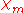(latin
Latin
Latin is an Italic language originally spoken in Latium and Ancient Rome. It, along with most European languages, is a descendant of the ancient Proto-Indo-European language. Although it is considered a dead language, a number of scholars and members of the Christian clergy speak it fluently, and...

: susceptibilis “receptiveness”) is a dimensionless proportionality constant that indicates the degree of magnetization
Magnetization
In classical electromagnetism, magnetization or magnetic polarization is the vector field that expresses the density of permanent or induced magnetic dipole moments in a magnetic material...

of a material in response to an applied magnetic field
Magnetic field
A magnetic field is a mathematical description of the magnetic influence of electric currents and magnetic materials. The magnetic field at any given point is specified by both a direction and a magnitude ; as such it is a vector field.Technically, a magnetic field is a pseudo vector;...

. A related term is magnetizability, the proportion between magnetic moment
Magnetic moment
The magnetic moment of a magnet is a quantity that determines the force that the magnet can exert on electric currents and the torque that a magnetic field will exert on it...

and magnetic flux density
Magnetic field
A magnetic field is a mathematical description of the magnetic influence of electric currents and magnetic materials. The magnetic field at any given point is specified by both a direction and a magnitude ; as such it is a vector field.Technically, a magnetic field is a pseudo vector;...

.

## Definition of volume susceptibility

The volume magnetic susceptibility, represented by the symbol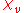(often simply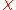, sometimes— magnetic, to distinguish from the electric susceptibility
Electric susceptibility
In electromagnetism, the electric susceptibility \chi_e is a dimensionless proportionality constant that indicates the degree of polarization of a dielectric material in response to an applied electric field...

), is defined by the relationship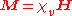where, in SI
International System of Units
The International System of Units is the modern form of the metric system and is generally a system of units of measurement devised around seven base units and the convenience of the number ten. The older metric system included several groups of units...

units,
M is the magnetization
Magnetization
In classical electromagnetism, magnetization or magnetic polarization is the vector field that expresses the density of permanent or induced magnetic dipole moments in a magnetic material...

of the material (the magnetic dipole moment per unit volume), measured in ampere
Ampere
The ampere , often shortened to amp, is the SI unit of electric current and is one of the seven SI base units. It is named after André-Marie Ampère , French mathematician and physicist, considered the father of electrodynamics...

s per meter, and

H is the magnetic field strength, also measured in amperes per meter.

The magnetic induction
Magnetic field
A magnetic field is a mathematical description of the magnetic influence of electric currents and magnetic materials. The magnetic field at any given point is specified by both a direction and a magnitude ; as such it is a vector field.Technically, a magnetic field is a pseudo vector;...

B is related to H by the relationship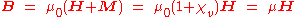where μ0 is the magnetic constant (see table of physical constant
Physical constant
A physical constant is a physical quantity that is generally believed to be both universal in nature and constant in time. It can be contrasted with a mathematical constant, which is a fixed numerical value but does not directly involve any physical measurement.There are many physical constants in...

s), and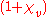is the relative permeability of the material.
Thus the volume magnetic susceptibilityand the magnetic permeability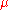are related by the following formula: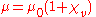.
Sometimes an auxiliary quantity, called intensity of magnetization (also referred to as magnetic polarisation J) and measured in teslas
Tesla (unit)
The tesla is the SI derived unit of magnetic field B . One tesla is equal to one weber per square meter, and it was defined in 1960 in honour of the inventor, physicist, and electrical engineer Nikola Tesla...

, is defined as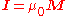.
This allows an alternative description of all magnetization phenomena in terms of the quantities I and B, as opposed to the commonly used M and H.

## Conversion between SI and CGS units

Note that these definitions are according to SI
International System of Units
The International System of Units is the modern form of the metric system and is generally a system of units of measurement devised around seven base units and the convenience of the number ten. The older metric system included several groups of units...

conventions. However, many tables of magnetic susceptibility give CGS
Centimetre gram second system of units
The centimetre–gram–second system is a metric system of physical units based on centimetre as the unit of length, gram as a unit of mass, and second as a unit of time...

values (more specifically emu-cgs, short for electromagnetic units, or Gaussian-cgs
Gaussian units
Gaussian units comprise a metric system of physical units. This system is the most common of the several electromagnetic unit systems based on cgs units. It is also called the Gaussian unit system, Gaussian-cgs units, or often just cgs units...

; both are the same in this context) that rely on a different definition of the permeability of free space: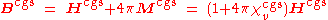The dimensionless CGS value of volume susceptibility is multiplied by 4π to give the dimensionless SI
International System of Units
The International System of Units is the modern form of the metric system and is generally a system of units of measurement devised around seven base units and the convenience of the number ten. The older metric system included several groups of units...

volume susceptibility value: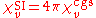For example, the CGS
Centimetre gram second system of units
The centimetre–gram–second system is a metric system of physical units based on centimetre as the unit of length, gram as a unit of mass, and second as a unit of time...

volume magnetic susceptibility of water at 20°C is −7.19×10−7 which is −9.04×10−6 using the SI
International System of Units
The International System of Units is the modern form of the metric system and is generally a system of units of measurement devised around seven base units and the convenience of the number ten. The older metric system included several groups of units...

convention.

## Mass susceptibility and molar susceptibility

There are two other measures of susceptibility, the mass magnetic susceptibilitymass or χg, sometimes χm), measured in m3·kg−1 in SI or in cm3·g−1 in CGS and the molar magnetic susceptibilitymol) measured in m3·mol−1 (SI) or cm3·mol−1 (CGS) that are defined below, where ρ is the density
Density
The mass density or density of a material is defined as its mass per unit volume. The symbol most often used for density is ρ . In some cases , density is also defined as its weight per unit volume; although, this quantity is more properly called specific weight...

in kg·m−3 (SI) or g·cm−3 (CGS) and M is molar mass
Molar mass
Molar mass, symbol M, is a physical property of a given substance , namely its mass per amount of substance. The base SI unit for mass is the kilogram and that for amount of substance is the mole. Thus, the derived unit for molar mass is kg/mol...

in kg·mol−1 (SI) or g·mol−1 (CGS).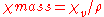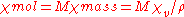## Sign of susceptibility: diamagnetics and other types of magnetism

If χ is positive, the material can be paramagnetic . In this case, the magnetic field in the material is strengthened by the induced magnetization. Alternatively, if χ is negative, the material is diamagnetic. As a result, the magnetic field in the material is weakened by the induced magnetization. Generally, non-magnetic materials are said para- or diamagnetic because they do not possess permanent magnetization without external magnetic field. Ferromagnetic, ferrimagnetic
Ferrimagnetism
In physics, a ferrimagnetic material is one in which the magnetic moments of the atoms on different sublattices are opposed, as in antiferromagnetism; however, in ferrimagnetic materials, the opposing moments are unequal and a spontaneous magnetization remains...

, or antiferromagnetic materials, which have positive susceptibility, possess permanent magnetization even without external magnetic field.

## Experimental methods to determine susceptibility

Volume magnetic susceptibility is measured by the force change felt upon the application of a magnetic field gradient. Early measurements were made using the Gouy balance where a sample is hung between the poles of an electromagnet. The change in weight when the electromagnet is turned on is proportional to the susceptibility. Today, high-end measurement systems use a superconductive magnet. An alternative is to measure the force change on a strong compact magnet upon insertion of the sample. This system, widely used today, is called the Evans balance
Evans balance
An Evans balance is a device for measuring magnetic susceptibility. Magnetic susceptibility is related to the force experienced by a substance in a magnetic field...

. For liquid samples, the susceptibility can be measured from the dependence of the NMR
Nuclear magnetic resonance
Nuclear magnetic resonance is a physical phenomenon in which magnetic nuclei in a magnetic field absorb and re-emit electromagnetic radiation...

frequency of the sample on its shape or orientation.

## Tensor susceptibility

The magnetic susceptibility of most crystal
Crystal
A crystal or crystalline solid is a solid material whose constituent atoms, molecules, or ions are arranged in an orderly repeating pattern extending in all three spatial dimensions. The scientific study of crystals and crystal formation is known as crystallography...

s is not a scalar. Magnetic response M is dependent upon the orientation of the sample and can occur in directions other than that of the applied field H. In these cases, volume susceptibility is defined as a tensor
Tensor
Tensors are geometric objects that describe linear relations between vectors, scalars, and other tensors. Elementary examples include the dot product, the cross product, and linear maps. Vectors and scalars themselves are also tensors. A tensor can be represented as a multi-dimensional array of...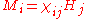where i and j refer to the directions (e.g., x and y in Cartesian coordinates) of the applied field and magnetization, respectively. The tensor
Tensor
Tensors are geometric objects that describe linear relations between vectors, scalars, and other tensors. Elementary examples include the dot product, the cross product, and linear maps. Vectors and scalars themselves are also tensors. A tensor can be represented as a multi-dimensional array of...

is thus rank 2 (second order), dimension (3,3) describing the component of magnetization in the i-th direction from the external field applied in the j-th direction.

## Differential susceptibility

In ferromagnetic crystals, the relationship between M and H is not linear. To accommodate this, a more general definition of differential susceptibility is used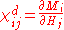where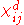is a tensor
Tensor
Tensors are geometric objects that describe linear relations between vectors, scalars, and other tensors. Elementary examples include the dot product, the cross product, and linear maps. Vectors and scalars themselves are also tensors. A tensor can be represented as a multi-dimensional array of...

derived from partial derivative
Partial derivative
In mathematics, a partial derivative of a function of several variables is its derivative with respect to one of those variables, with the others held constant...

s of components of M with respect to components of H.
When the coercivity
Coercivity
In materials science, the coercivity, also called the coercive field or coercive force, of a ferromagnetic material is the intensity of the applied magnetic field required to reduce the magnetization of that material to zero after the magnetization of the sample has been driven to saturation...

of the material parallel to an applied field is the smaller of the two, the differential susceptibility is a function of the applied field and self interactions, such as the magnetic anisotropy
Magnetic anisotropy
Magnetic anisotropy is the direction dependence of a material's magnetic properties. In the absence of an applied magnetic field, a magnetically isotropic material has no preferential direction for its magnetic moment while a magnetically anisotropic material will align its moment with one of the...

. When the material is not saturated, the effect will be nonlinear and dependent upon the domain wall
Domain wall
A domain wall is a term used in physics which can have one of two distinct but similar meanings in magnetism, optics, or string theory. These phenomena can all be generically described as topological solitons which occur whenever a discrete symmetry is spontaneously broken.-Magnetism:In magnetism,...

configuration of the material.

## Susceptibility in the frequency domain

When the magnetic susceptibility is measured in response to an AC
Alternating current
In alternating current the movement of electric charge periodically reverses direction. In direct current , the flow of electric charge is only in one direction....

magnetic field (i.e. a magnetic field that varies sinusoidally), this is called AC susceptibility. AC susceptibility (and the closely related "AC permeability") are complex
Complex number
A complex number is a number consisting of a real part and an imaginary part. Complex numbers extend the idea of the one-dimensional number line to the two-dimensional complex plane by using the number line for the real part and adding a vertical axis to plot the imaginary part...

quantities, and various phenomena (such as resonances) can be seen in AC susceptibility that cannot in constant-field (DC) susceptibility. In particular, when an ac-field is applied perpendicular to the detection direction (called the "transverse susceptibility" regardless of the frequency), the effect has a peak at the ferromagnetic resonance
Ferromagnetic resonance
Ferromagnetic resonance, or FMR, is a spectroscopic technique to probe the magnetization of ferromagnetic materials. It is a standard tool for probing spin waves and spin dynamics...

frequency of the material with a given static applied field. Currently, this effect is called the microwave permeability or network ferromagnetic resonance in the literature. These results are sensitive to the domain wall
Domain wall
A domain wall is a term used in physics which can have one of two distinct but similar meanings in magnetism, optics, or string theory. These phenomena can all be generically described as topological solitons which occur whenever a discrete symmetry is spontaneously broken.-Magnetism:In magnetism,...

configuration of the material and eddy currents.

In terms of ferromagnetic resonance
Ferromagnetic resonance
Ferromagnetic resonance, or FMR, is a spectroscopic technique to probe the magnetization of ferromagnetic materials. It is a standard tool for probing spin waves and spin dynamics...

, the effect of an ac-field applied along the direction of the magnetization is called parallel pumping.

## Examples

Magnetic susceptibility of some materials
Material Temperature
Temperature
Temperature is a physical property of matter that quantitatively expresses the common notions of hot and cold. Objects of low temperature are cold, while various degrees of higher temperatures are referred to as warm or hot...

Pressure
Pressure
Pressure is the force per unit area applied in a direction perpendicular to the surface of an object. Gauge pressure is the pressure relative to the local atmospheric or ambient pressure.- Definition :...

|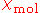(molar susc.)|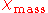(mass susc.) |(volume susc.) M (molar mass
Molar mass
Molar mass, symbol M, is a physical property of a given substance , namely its mass per amount of substance. The base SI unit for mass is the kilogram and that for amount of substance is the mole. Thus, the derived unit for molar mass is kg/mol...

)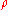(density
Density
The mass density or density of a material is defined as its mass per unit volume. The symbol most often used for density is ρ . In some cases , density is also defined as its weight per unit volume; although, this quantity is more properly called specific weight...

)
Units
Units of measurement
A unit of measurement is a definite magnitude of a physical quantity, defined and adopted by convention and/or by law, that is used as a standard for measurement of the same physical quantity. Any other value of the physical quantity can be expressed as a simple multiple of the unit of...

(°C
Celsius
Celsius is a scale and unit of measurement for temperature. It is named after the Swedish astronomer Anders Celsius , who developed a similar temperature scale two years before his death...

)
(atm
Atmosphere (unit)
The standard atmosphere is an international reference pressure defined as 101325 Pa and formerly used as unit of pressure. For practical purposes it has been replaced by the bar which is 105 Pa...

)
SI
(m3·mol
Mole (unit)
The mole is a unit of measurement used in chemistry to express amounts of a chemical substance, defined as an amount of a substance that contains as many elementary entities as there are atoms in 12 grams of pure carbon-12 , the isotope of carbon with atomic weight 12. This corresponds to a value...

−1)
CGS
(cm3·mol
Mole (unit)
The mole is a unit of measurement used in chemistry to express amounts of a chemical substance, defined as an amount of a substance that contains as many elementary entities as there are atoms in 12 grams of pure carbon-12 , the isotope of carbon with atomic weight 12. This corresponds to a value...

−1)
SI
(m3·kg−1)
CGS
(cm3·g
Gram
The gram is a metric system unit of mass....

−1)
SI
CGS
(emu)
(10−3 kg/mol
Mole (unit)
The mole is a unit of measurement used in chemistry to express amounts of a chemical substance, defined as an amount of a substance that contains as many elementary entities as there are atoms in 12 grams of pure carbon-12 , the isotope of carbon with atomic weight 12. This corresponds to a value...

)
or (g
Gram
The gram is a metric system unit of mass....

/mol
Mole (unit)
The mole is a unit of measurement used in chemistry to express amounts of a chemical substance, defined as an amount of a substance that contains as many elementary entities as there are atoms in 12 grams of pure carbon-12 , the isotope of carbon with atomic weight 12. This corresponds to a value...

)
(103 kg/m3)
or (g
Gram
The gram is a metric system unit of mass....

/cm3)
vacuum
Vacuum
In everyday usage, vacuum is a volume of space that is essentially empty of matter, such that its gaseous pressure is much less than atmospheric pressure. The word comes from the Latin term for "empty". A perfect vacuum would be one with no particles in it at all, which is impossible to achieve in...

Any 0 0 0 0 0 0 0 0
water
Water (data page)
This page provides supplementary data of the properties of water.Further comprehensive authoritative data can be found at the page on thermophysical properties of fluids.-Structure and properties:-Thermodynamic properties:-Liquid physical properties:...

20 1 −1.631×10−10 −1.298×10−5 −9.051×10−9 −7.203×10−7 −9.035×10−6 −7.190×10−7 18.015 0.9982
bismuth
Bismuth
Bismuth is a chemical element with symbol Bi and atomic number 83. Bismuth, a trivalent poor metal, chemically resembles arsenic and antimony. Elemental bismuth may occur naturally uncombined, although its sulfide and oxide form important commercial ores. The free element is 86% as dense as lead...

20 1 −3.55×10−9 −2.82×10−4 −1.70×10−8 −1.35×10−6 −1.66×10−4 −1.32×10−5 208.98 9.78
Diamond
Carbon
Carbon is the chemical element with symbol C and atomic number 6. As a member of group 14 on the periodic table, it is nonmetallic and tetravalent—making four electrons available to form covalent chemical bonds...

R.T.
Room temperature
-Comfort levels:The American Society of Heating, Refrigerating and Air-Conditioning Engineers has listings for suggested temperatures and air flow rates in different types of buildings and different environmental circumstances. For example, a single office in a building has an occupancy ratio per...

1 −7.4×10−11 −5.9×10−6 −6.2×10−9 −4.9×10−7 −2.2×10−5 −1.7×10−6 12.01 3.513
Graphite
Carbon
Carbon is the chemical element with symbol C and atomic number 6. As a member of group 14 on the periodic table, it is nonmetallic and tetravalent—making four electrons available to form covalent chemical bonds...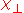(to c-axis)
R.T.
Room temperature
-Comfort levels:The American Society of Heating, Refrigerating and Air-Conditioning Engineers has listings for suggested temperatures and air flow rates in different types of buildings and different environmental circumstances. For example, a single office in a building has an occupancy ratio per...

1 −7.5×10−11 −6.0×10−6 −6.3×10−9 −5.0×10−7 −1.4×10−5 −1.1×10−6 12.01 2.267
Graphite
Carbon
Carbon is the chemical element with symbol C and atomic number 6. As a member of group 14 on the periodic table, it is nonmetallic and tetravalent—making four electrons available to form covalent chemical bonds...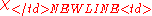R.T.
Room temperature
-Comfort levels:The American Society of Heating, Refrigerating and Air-Conditioning Engineers has listings for suggested temperatures and air flow rates in different types of buildings and different environmental circumstances. For example, a single office in a building has an occupancy ratio per...

1 −3.2×10−9 −2.6×10−4 −2.7×10−7 −2.2×10−5 −6.1×10−4 −4.9×10−5 12.01 2.267
Graphite
Carbon
Carbon is the chemical element with symbol C and atomic number 6. As a member of group 14 on the periodic table, it is nonmetallic and tetravalent—making four electrons available to form covalent chemical bonds...-173 1 −4.4×10−9 −3.5×10−4 −3.6×10−7 −2.9×10−5 −8.3×10−4 −6.6×10−5 12.01 2.267
He
Helium
Helium is the chemical element with atomic number 2 and an atomic weight of 4.002602, which is represented by the symbol He. It is a colorless, odorless, tasteless, non-toxic, inert, monatomic gas that heads the noble gas group in the periodic table...

20 1 −2.38×10−11 −1.89×10−6 −5.93×10−9 −4.72×10−7 −9.85×10−10 −7.84×10−11 4.0026 0.000166
Xe
Xenon
Xenon is a chemical element with the symbol Xe and atomic number 54. The element name is pronounced or . A colorless, heavy, odorless noble gas, xenon occurs in the Earth's atmosphere in trace amounts...

20 1 −5.71×10−10 −4.54×10−5 −4.35×10−9 −3.46×10−7 −2.37×10−8 −1.89×10−9 131.29 0.00546
O2
Oxygen
Oxygen is the element with atomic number 8 and represented by the symbol O. Its name derives from the Greek roots ὀξύς and -γενής , because at the time of naming, it was mistakenly thought that all acids required oxygen in their composition...

20 0.209 4.3×10−8 3.42×10−3 1.34×10−6 1.07×10−4 3.73×10−7 2.97×10−8 31.99 0.000278
N2
Nitrogen
Nitrogen is a chemical element that has the symbol N, atomic number of 7 and atomic mass 14.00674 u. Elemental nitrogen is a colorless, odorless, tasteless, and mostly inert diatomic gas at standard conditions, constituting 78.08% by volume of Earth's atmosphere...

20 0.781 −1.56×10−10 −1.24×10−5 −5.56×10−9 −4.43×10−7 −5.06×10−9 −4.03×10−10 28.01 0.000910
Al
Aluminium
Aluminium or aluminum is a silvery white member of the boron group of chemical elements. It has the symbol Al, and its atomic number is 13. It is not soluble in water under normal circumstances....

1 2.2×10−10 1.7×10−5 7.9×10−9 6.3×10−7 2.2×10−5 1.75×10−6 26.98 2.70
Ag
Silver
Silver is a metallic chemical element with the chemical symbol Ag and atomic number 47. A soft, white, lustrous transition metal, it has the highest electrical conductivity of any element and the highest thermal conductivity of any metal...

961 1 −2.31×10−5 −1.84×10−6 107.87

## Sources of confusion in published data

There are tables of magnetic susceptibility values published on-line that seem to have been uploaded from a substandard source,
which itself has probably borrowed heavily from the CRC Handbook of Chemistry and Physics
CRC Press
The CRC Press, LLC is a publishing group which specializes in producing technical books. While many of their books relate to engineering, science and mathematics, their scope also includes books on business, forensics and information technology...

. Some of the data (e.g. for Al, Bi, and diamond) are apparently in cgs Molar Susceptibility units, whereas that for water is in Mass Susceptibility units (see discussion above). The susceptibility table in the CRC Handbook is known to suffer from similar errors, and even to contain sign errors. Effort should be made to trace the data in such tables to the original sources, and to double-check the proper usage of units.

• Curie constant
• Electric susceptibility
Electric susceptibility
In electromagnetism, the electric susceptibility \chi_e is a dimensionless proportionality constant that indicates the degree of polarization of a dielectric material in response to an applied electric field...

• Iron
Iron
Iron is a chemical element with the symbol Fe and atomic number 26. It is a metal in the first transition series. It is the most common element forming the planet Earth as a whole, forming much of Earth's outer and inner core. It is the fourth most common element in the Earth's crust...

• Magnetic constant
• Magnetic flux density
• Magnetism
Magnetism
Magnetism is a property of materials that respond at an atomic or subatomic level to an applied magnetic field. Ferromagnetism is the strongest and most familiar type of magnetism. It is responsible for the behavior of permanent magnets, which produce their own persistent magnetic fields, as well...

• Magnetochemistry
Magnetochemistry
Magnetochemistry is concerned with the magnetic properties of chemical compounds. Magnetic properties arise from the spin and orbital angular momentum of the electrons contained in a compound. Compounds are diamagnetic when they contain no unpaired electrons. Molecular compounds that contain one or...

• Magnetometer
Magnetometer
A magnetometer is a measuring instrument used to measure the strength or direction of a magnetic field either produced in the laboratory or existing in nature...

• Maxwell's equations
Maxwell's equations
Maxwell's equations are a set of partial differential equations that, together with the Lorentz force law, form the foundation of classical electrodynamics, classical optics, and electric circuits. These fields in turn underlie modern electrical and communications technologies.Maxwell's equations...

• Paleomagnetism
Paleomagnetism
Paleomagnetism is the study of the record of the Earth's magnetic field in rocks. Certain minerals in rocks lock-in a record of the direction and intensity of the magnetic field when they form. This record provides information on the past behavior of Earth's magnetic field and the past location of...

• Permeability (electromagnetism)
Permeability (electromagnetism)
In electromagnetism, permeability is the measure of the ability of a material to support the formation of a magnetic field within itself. In other words, it is the degree of magnetization that a material obtains in response to an applied magnetic field. Magnetic permeability is typically...

• Susceptibility weighted imaging
Susceptibility weighted imaging
Susceptibility weighted imaging , originally called BOLD venographic imaging, uses a type of contrast in magnetic resonance imaging different from traditional spin density, T1, or T2 imaging. SWI uses a fully flow compensated, long echo, gradient echo scan to acquire images...